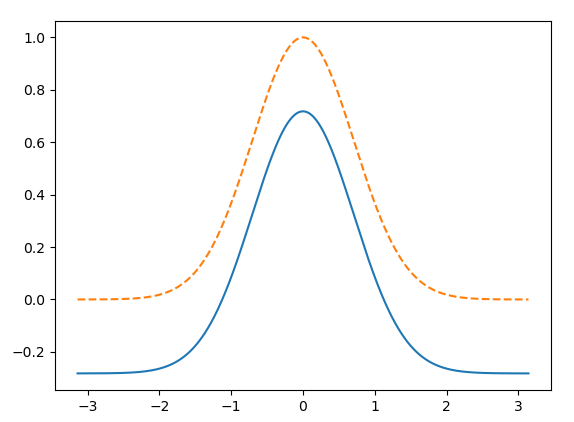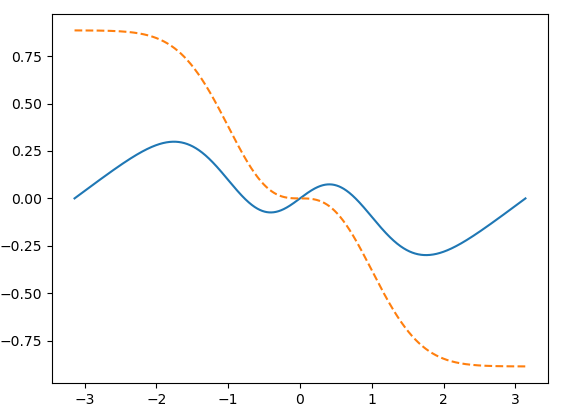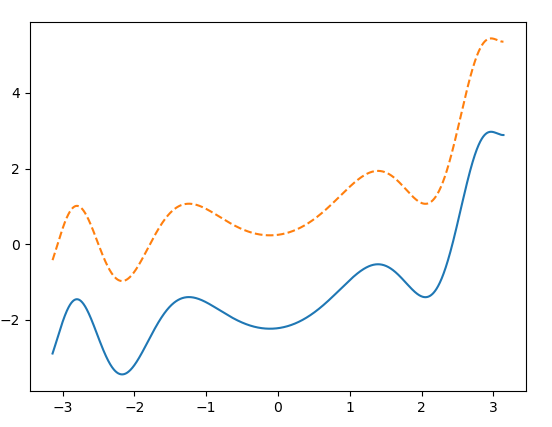# Is there a way to numerically integrate in Fourier space with scipy.fft?

I am interested in integrating in Fourier space after using scipy to take an fft of some data. I have been following along with this stack exchange post numerical integration in Fourier space with numpy.fft but it does not properly integrate a few test cases I have been working with. I have added a few lines to address this issue but still am not recovering the correct integrals. Below is the code I have been using to integrate my test cases. At the top of the code are the 3 test cases I have been using.

``````import numpy as np
import scipy.special as sp
from scipy.fft import fft, ifft, fftfreq
import matplotlib.pyplot as plt

#set number of points in array
Ns = 2**16
#create array in space
x = np.linspace(-np.pi, np.pi, Ns)

#test case 1 from stack exchange post
# y = np.exp(-x**2)                          # function f(x)
# ys = np.exp(-x**2) * (-2 *x)               # derivative f'(x)

#test case 2
# y = np.exp(-x**2) * x - 1/2 *np.sqrt(np.pi)*sp.erf(x)
# ys =  np.exp(-x**2) * -2*x**2

#test case 3
y = np.sin(x**2) + (1/4)*np.exp(x)
ys = 1/4*(np.exp(x) + 8*x*np.cos(x**2))

#find spacing in space array
ss = x-x

#definte fft integration function
def fft_int(N,s,dydt):
#create frequency array
f = fftfreq(N,s)

# integration step ignoring divide by 0 errors
Fys = fft(dydt)
with np.errstate(divide="ignore", invalid="ignore"):
modFys = Fys / (2*np.pi*1j*f)

#set DC term to 0, was a nan since we divided by 0
modFys = 0

#take inverse fft and subtract by integration constant
fourier = ifft(modFys)
fourier = fourier-fourier

#tilt correction if function doesn't approach 0 at its ends
tilt = np.sum(dydt)*s*(np.arange(0,N)/(N-1) - 1/2)
fourier = fourier + tilt

return fourier
``````

Test case 1 was from the stack exchange post from above. If you copy paste the code from the top answer and plot you'll get something like this:with the solid blue line being the fft integration method and the dashed orange as the analytic solution. I account for this offset with the following line of code:

``````fourier = fourier-fourier
``````

since I don't believe the code was setting the constant of integration.

Next for test case 2 I get a plot like this:again with the solid blue line being the fft integration method and the dashed orange as the analytic solution. I account for this tilt in the solution using the following lines of code

``````tilt = np.sum(dydt)*s*(np.arange(0,N)/(N-1) - 1/2)
fourier = fourier + tilt
``````

Finally we arrive at test case 3. Which results in the following plot:again with the solid blue line being the fft integration method and the dashed orange as the analytic solution. This is where I'm stuck, this offset has appeared again and I'm not sure why.

TLDR: How do I correctly integrate a function in fourier space using scipy.fft?